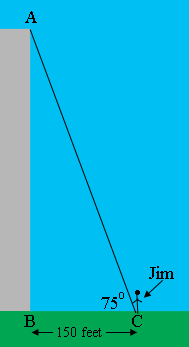SEARCH HOMEMath Central Quandaries & QueriesQuestion from Jim: If I'm 150 ft. from the base of a building and the top of the building is approx. at a 75 degree angle, how do you calculate the height?Hi Jim,

I drew a diagram of you, 150 feet from the base of the building. The height of the building is the length of $AB$ in the diagram.What trig function relates the measure of the angle $BCA$ and the lengths of the sides $AB$ and $BC?$

PennyMath Central is supported by the University of Regina and the Imperial Oil Foundation.